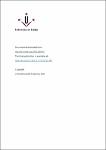## Modified inertial mass from information loss2017
McCulloch, M. E.
##### Abstract
A modification of inertia (called MiHsC or quantized inertia) has been proposed that assumes that inertia is caused by Unruh radiation, and that this radiation is made inhomogeneous in space by either Rindler horizons caused by acceleration or the distant Hubble horizon. The former predicts the standard inertial mass, and the latter predicts galaxy rotation without dark matter and cosmic acceleration without dark energy. It is proposed here that this model can be derived in an alternative way by assuming that the sum of mass (M), energy (E) and the information content of horizons (I) is conserved (EMI) so that mass–energy is released in a discrete manner when the area of a Rindler horizon reduces. This model could be tested by looking for the quantization of inertial mass and acceleration at very high accelerations, and may provide an explanation for the cosmological constant problem.
##### Journal or Serie
Modern Physics Letters A, 2017, vol. 32, núm. 28, a1750148